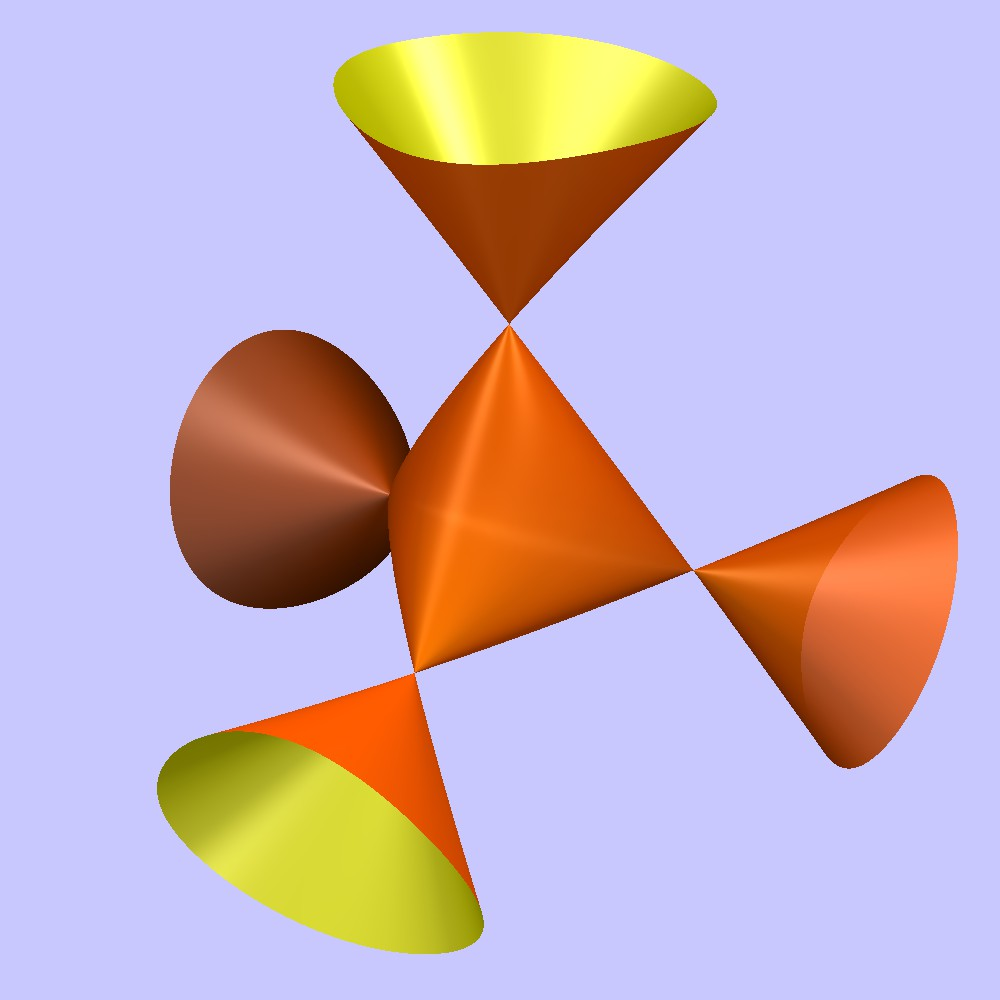# Nodal Cubic

A nodal curve is any curve with a node (i.e., a nodal singularity). The “usual” ℝ2 (real-valued) nodal cubic is defined by the equation 

y2x2(x + 1) = 0,

or equivalently

y2x3x2 = 0.

Like all cubics, the nodal curve is defined by a polynomial of degree 3.

For complex-valued equations, the nodal cubic is a limit of elliptic curves.

y2 = x(x + ε)(x – 1).

## Properties of the Nodal Cubic

• The usual form has one double point at the origin, where the curve crosses over itself.
• Tangents are at the node along the x- and y-axes.
• The curve is homeomorphic to a figure 8 (i.e., it has a one-to-one continuous mapping in both directions).
• It isn’t a union of other curves, so it is irreducible (the polynomials that define the cubic are by definition, also irreducible).
• Nodal cubics are nondegenerate, meaning that they cannot be expressed as a finite union of conics, lines, and points .
• The cubic is a member of the Folium class of curves under projective equivalence. However, it belongs to a separate class under affine equivalence .

## Cayley’s Nodal CubicCayley’s nodal cubic surface has four double points, which is the largest possible number of double points; every cubic with four double points is isomorphic to Cayley’s surface. The surface is defined by the equation 

wxy + wxz + wyz + xyz = 0.

## References

Cayley’s cubic image: Salix alba, CC BY-SA 3.0, via Wikimedia Commons.
 Desmos.com. Nodal.
 Nodal and cuspidal curves. Retrieved March 3, 2022 from: https://www.math.purdue.edu/~arapura/graph/nodal.html
 Ding, A. Plane Algebraic Curves. Retrieved March 3, 2022 from: https://staff.math.su.se/shapiro/UIUC/DingPlaneCurves.pdf
 Holme, A. A Royal Road to Algebraic Geometry. Springer Berlin Heidelberg.
 Baez, J. (2016). Cayley’s Nodal Cubic Surface. Retrieved March 3, 2022 from: https://blogs.ams.org/visualinsight/2016/08/15/cayleys-nodal-cubic-surface/

CITE THIS AS:
Stephanie Glen. "Nodal Cubic" From StatisticsHowTo.com: Elementary Statistics for the rest of us! https://www.statisticshowto.com/nodal-cubic/